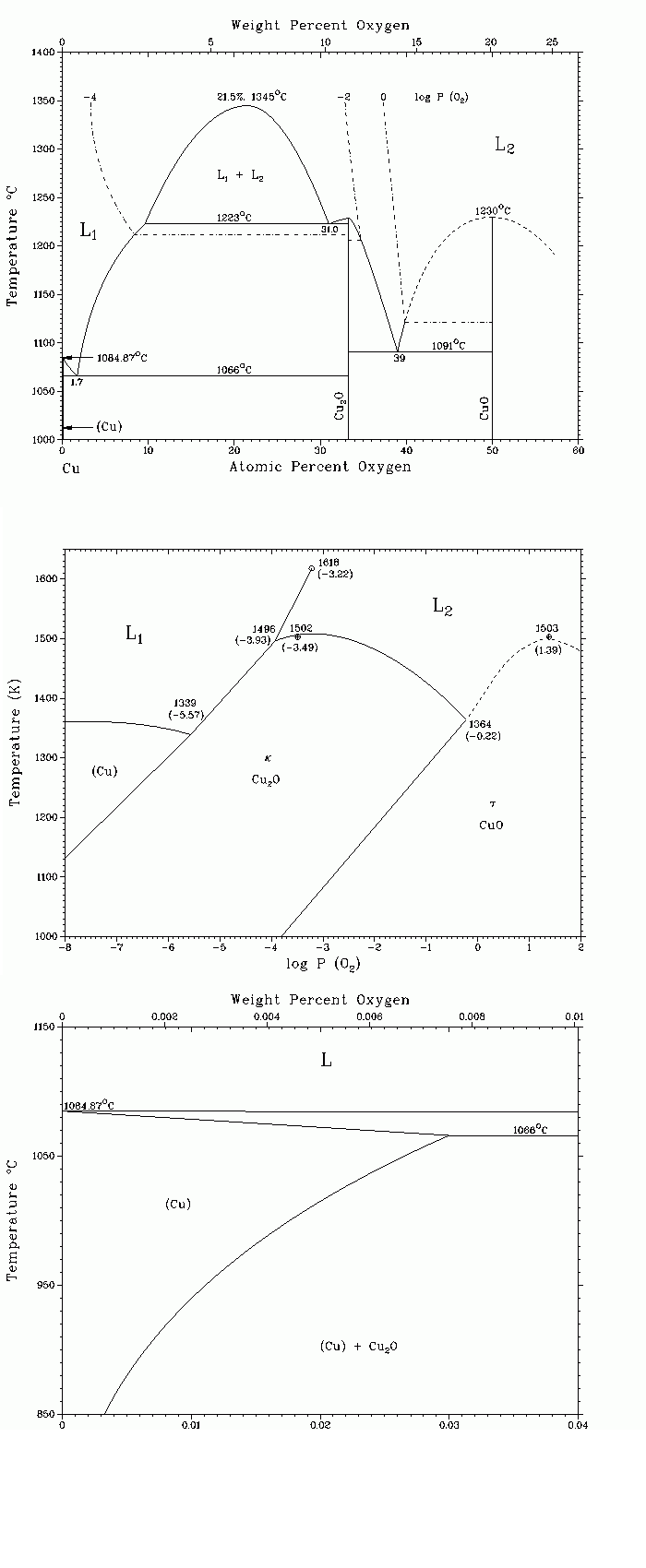# Фазовая диаграмма системы Cu-OCu-O (Copper-Oxygen) J.P. Neumann, T. Zhong, and Y.A. Chang The assessed Cu-O phase diagram and the Cu-O stability diagram were synthesized from [Metals], [76Ell], and [83Sch]. The phase diagram in [Hansen] indicated a much larger liquid miscibility gap than the present diagram. This discrepancy is due to the fact that oxygen-rich Cu melts dissolve many refractory oxides that are used as crucible materials, resulting in a larger miscibility gap. Earlier investigators were unaware of this problem. The solubility of oxygen in solid Cu is very small and reaches a maximum at the eutectic temperature. According to [Metals], the solubility is 0.015 at.% O at this temperature. Three intermediate phases exist: Cu2O, Cu4O3, and CuO. In addition to the condensed solid and liquid phases, a gas phase, oxygen, also exists. For a complete description of the system, it is therefore necessary to specify not only the composition and temperature of the condensed phases, but also the corresponding equilibrium pressure of oxygen in the gas phase. This is done partially in the assessed phase diagram by indicating some isobars corresponding to oxygen pressures of log ( po2/bar) = -4, -2, and 0. The solubility limit of oxygen in the metal-rich liquid (L1) at three points- the eutectic point, 1066 C (1339 K), 1.7 at.% O; the Cu-rich end of the monotectic invariant, 1223 C (1496 K), 9.7 at.% O; and the critical point of the liquid miscibility gap, 1345 C (1618 K), 21.5 at.% O-were calculated, and the following values for log ( po2/bar) were obtained, respectively, -5.63, -3. 94, and -3.26. Good agreement was found with the phase diagram. On heating CuO in air (log po2/bar = -0.67), it dissociates at 1304 K to form Cu2O, which in turn melts incongruently at 1407 K. Under an oxygen pressure of 1 bar (log po2/bar = 0), CuO melts incongruently at 1394 K [76Ell]; congruent melting of CuO occurs at 1503 K under an oxygen pressure of 24.7 bar (log po2/ bar = +1.39) [83Sch]. 76Ell: J.F. Elliot, Metall. Trans. B, 7, 17-33 (1976). 83Sch: R. Schmid, Metall. Trans. B, 14, 473-481 (1983). Published in Bull. Alloy Phase Diagrams, 5(2), Apr 1984. Complete evaluation contains 4 figures, 2 tables, and 46 references. Special Points of the Cu-O System